Select Page

Computer Organization

Memory Organization
Interrupt
DMA Controller

# Laws of Boolean Algebra

According to laws of Boolean Algebra , we use some variables i.e. (A, B, C, X, Y, Z) and Apply NOT, AND, OR operations on these variables.

## Types of Laws in Boolean Algebra

There are several laws of Boolean algebra which are given below, let explain all equations of these laws with proof through Truth Table.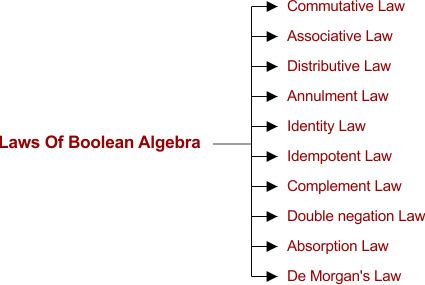### 1.Commutative Law

According to commutative law

• Any order in which two variables are AND’ed makes the similar result always (i.e. A . B = B . A )
• Any order in which two variables are OR’ed makes the similar result always (i.e. A + B = B + A)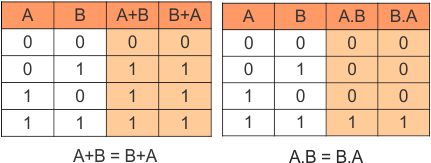### 2. Associative Law

• A.(B.C) = (A.B).C = A . B . C    (AND Associate Law)
• A + (B + C) = (A + B) + C = A + B + C    (OR Associate Law)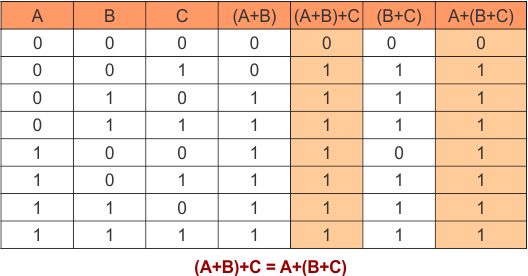### 3. Distributive Law

• A.(B + C) = (A.B) + (A.C)    (OR Distributive Law)
• A + (B.C) = (A + B) . (A + C)    (AND Distributive Law)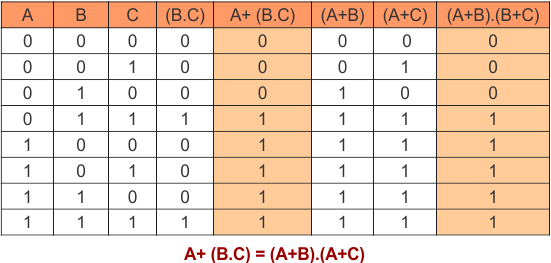### 4. Annulment law

According to Annulment Law

• A variable AND’ed with 0 will always be equal to 0 (i.e. A . 0 = 0)
• A variable OR’ed with 1 will always be equal to 1 (i.e.  A + 1 = 1)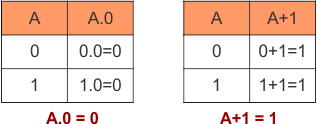### 5. Identity law

According to identity Law

• A variable OR’ed with 0 will always be equal to that variable (i.e. A + 0 = A)
• A variable AND’ed with 1 will always be equal to that variable (i.e. A . 1 = A)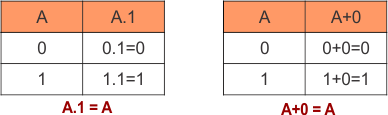### 6. Idempotent law

According to idempotent law

• A variable OR’ed with itself will always be equal to that variable (i.e. A + A = A)
• A variable AND’ed with itself will always be equal to that variable (i.e. A . A = A )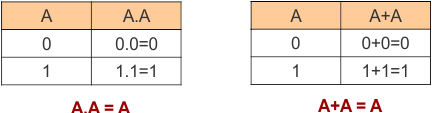### 7. Complement law

According to complement law

•   A variable AND’ed with its complement will always equal to 0.  (i.e. A . A’ = 0)
•  A variable OR’ed with its complement will always equal to 1. (i.e. A + A’ = 1)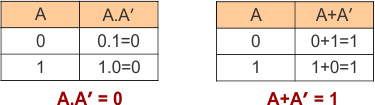### 8. Double Negation law

According to double negation law

• Double complement of a variable will always equal to that variable. (i.e. (A’)’ =A)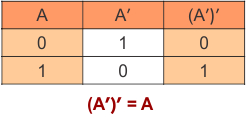### 9. Absorption law

• A + (A.B) = A  (OR Absorption Law)
• A . (A+B) = A  (AND Absorption Law)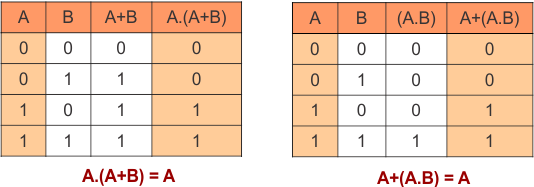### 10. De Morgan’s Law

According to De Morgan’s law

• (A.B)’ = A’ + B’
• (A+B)’ = A’ . B’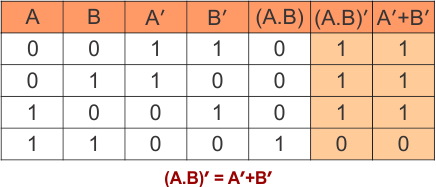Help Other’s By Sharing…